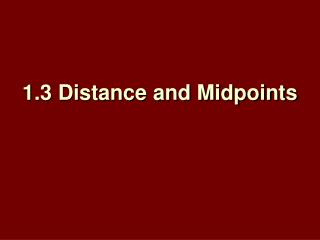# 1.3 Distance and Midpoints - PowerPoint PPT PresentationDownload Presentation1.3 Distance and Midpoints

1.3 Distance and MidpointsDownload Presentation## 1.3 Distance and Midpoints

- - - - - - - - - - - - - - - - - - - - - - - - - - - E N D - - - - - - - - - - - - - - - - - - - - - - - - - - -
##### Presentation Transcript

1. 1.3 Distance and Midpoints

2. Objectives: • Find the distance between two points using the distance formula and Pythagorean’s Theorem. • Find the midpoint of a segment.

3. In the last section we learned that whenever you connect two points you create a segment. We also learned every segment has a measure. The distancebetween two points, or the measure of a segment, is determined by the number of units between the two points. Distance Between Two Points

4. Distance Formula on a Number Line • If a segment is on a number line, we simply find its length by using the Distance Formula which states the distance between two points is the absolute value of the difference of the values of the two points. | A – B | = | B – A | = Distance

5. Example 1: Use the number line to find QR. The coordinates of Q and R are –6 and –3. Distance Formula Simplify. Answer: 3

6. Your Turn: Use the number line to find AX. Answer: 8

7. Distance Formula on a Coordinate Plane • Segments may also be drawn on coordinate planes. To find the distance between two points on a coordinate plane with coordinates (x1, y1) and (x2, y2) we can use this formula:

8. Distance Formula on a Coordinate Plane … or we can use the Pythagorean Theorem. The Pythagorean Theorem simply states that the square of the hypotenuse equals the sum of the squares of the two legs. a2 + b2 = c2

9. Example 2: Find the distance between E(–4, 1) and F(3, –1). Pythagorean Theorem Method Use the gridlines to form a triangle so you can use the Pythagorean Theorem.

10. Example 2: Pythagorean Theorem Simplify. Take the square root of each side.

11. Simplify. Answer: The distance from E to F is units. You can use a calculator to find that is approximately 7.28. Example 2: Distance Formula Method Distance Formula Simplify.

12. Answer: Your Turn: Find the distance between A(–3, 4) and M(1, 2).

13. Midpoint of a Segment • The midpoint of a segment is the point halfway between the endpoints of the segment. If X is the midpoint of AB, then AX XB. • To find the midpoint of a segment on a number line find ½ of the sum of the coordinates of the two endpoints. a + b 2

14. The coordinates on a number line of J and K are –12and 16, respectively. Find the coordinate of the midpoint of . Let M be the midpoint of . Example 3: The coordinates of J and K are –12 and 16. Simplify. Answer: 2

15. Midpoint of a Segment • If the segment is on a coordinate plane, we must use the midpoint formula for coordinate planeswhich states givena segment with endpoints (x1, y1) and(x2, y2) the midpoint is… M ( x1 + x2 , y1 + y2 ) 2 2

16. Find the coordinates ofM, the midpoint of ,forG(8, –6) andH(–14, 12). Let G be and H be . Example 4: - Answer: (–3, 3)

17. a. The coordinates on a number line of Y and O are 7 and –15, respectively. Find the coordinate of the midpoint of . b. Find the coordinates of the midpoint of for X(–2, 3) and Y(–8, –9). Your Turn: Answer: –4 Answer: (–5, –3)

18. More About Midpoints • You can also find the coordinates of an endpoint of a segment if you know the coordinates of the other endpoint and its midpoint.

19. Find the coordinates ofDifE(–6, 4) is the midpoint of andFhas coordinates (–5, –3). Let F be in the Midpoint Formula. Example 5: Write two equations to find the coordinates of D.

20. Example 5: Solve each equation. Multiply each side by 2. Add 5 to each side. Multiply each side by 2. Add 3 to each side. Answer: The coordinates of D are (–7, 11).

21. Find the coordinates ofRifN(8, –3) is the midpoint of andS has coordinates (–1, 5). Your Turn: Answer: (17, –11)

22. Multiple-Choice Test ItemWhat is the measure of ifQis the midpoint of ? AB 4 CD 9 Example 6:

23. You know that Q is the midpoint of , and the figure gives algebraic measures for and . You are asked to find the measure of . Because Q is the midpoint, you know that . Use this equation and the algebraic measures to find a value for x. Example 6: Read the Test Item Solve the Test Item

24. Example 6: Definition of midpoint Distributive Property Subtract 1 from each side. Add 3x to each side. Divide each side by 10.

25. Now substitute for x in the expression for PR. Example 6: Original measure Simplify. Answer: D

26. Multiple-Choice Test ItemWhat is the measure of ifBis the midpoint of ? A 1 B 3 C 5 D 10 Your Turn: Answer: B

27. Assignment: • Geometry: Pg. 25 – 26, #13 – 20, 23, 24, 31 – 38, 43, 44 • Pre-AP Geometry: Pg. 25 – 26, #14 – 44 evens